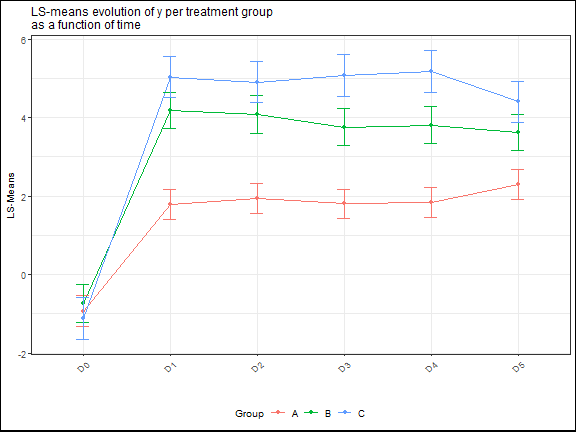## Objective

In this vignette we will see the different graphics that you can produce with ClinReport.

## First step

``````library(ClinReport)
library(officer)
library(flextable)
library(dplyr)
library(reshape2)
library(nlme)
library(emmeans)
library(car)
``````

``````# We will use fake data
data(datafake)
#>    y_numeric y_logistic y_poisson   baseline   VAR GROUP TIMEPOINT SUBJID
#> 1 -0.4203490          1         5 -0.4203490 Cat 1     A        D0 Subj 1
#> 2 -0.1570941          1         5 -0.1570941 Cat 2     A        D0 Subj 1
#> 3         NA          0         3 -2.0853720 Cat 2     A        D0 Subj 1
#> 4 -0.4728527          0         5 -0.4728527 Cat 1     A        D0 Subj 1
#> 5 -0.8651713          1         4 -0.8651713 Cat 1     A        D0 Subj 1
#> 6 -1.5476907          1         3 -1.5476907 Cat 1     A        D0 Subj 1
``````

### Quantitative graphics

First you need to produce a quantitative table using `report.quanti()` function then you use the `plot()` function on the result:

If there is no explicative variable, the corresponding graphic of the call to `report.quanti()` is a barplot of the mean of the response value.

``````# Only one numerical response

tab1=report.quanti(data=datafake,y="y_numeric")
plot(tab1,title="Mean (+/-SD) of y",add.sd=T,ylab="Mean Response value",xlab="")
``````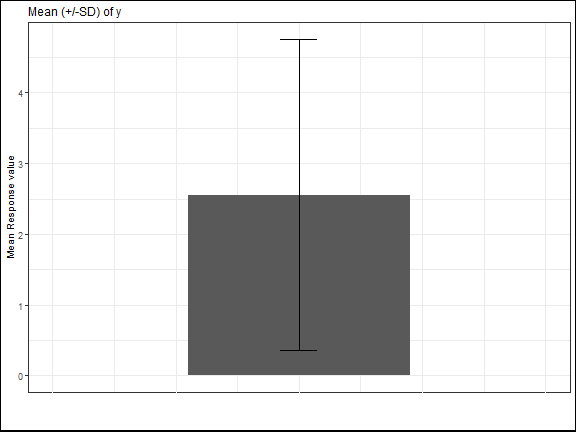If there is one explicative variable, the corresponding graphic will be a barplot per treatment group.

You can personnalize the outputs using, the usual, `title` `xlab` `ylab` `ylim` etc.. options.

``````#  one numerical response ; one explicative variable

tab2=report.quanti(data=datafake,y="y_numeric",x1="GROUP")
plot(tab2,title="Mean (+/-SD) of y per treatment group",add.sd=T,ylab="Mean of y",xlab="",
ylim=c(-1,8))
``````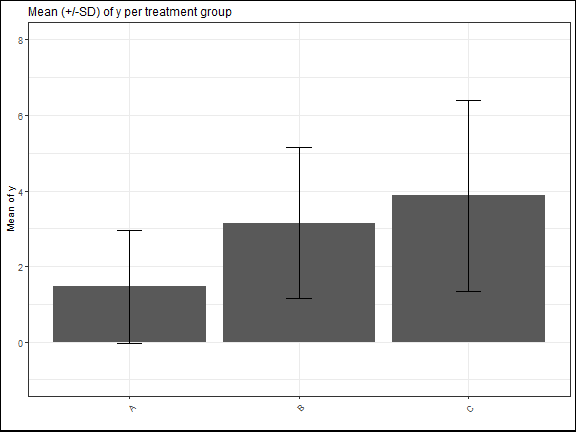If there is also a second explicative variable. The corresponding plot will be a lineplot:

The means will be connected according to this second variable to capture the evolution across its different levels.

``````#  one numerical response ; two explicative variable

tab3=report.quanti(data=datafake,y="y_numeric",x1="GROUP",x2="TIMEPOINT")
plot(tab3,title="Mean (+/-SD) of y per treatment group",add.sd=T,ylab="Mean of y",xlab="",
ylim=c(-1,8))
#> Warning: Removed 1 rows containing missing values (geom_point).
#> Warning: Removed 1 rows containing missing values (geom_path).
#> Warning: Removed 3 rows containing missing values (geom_errorbar).
``````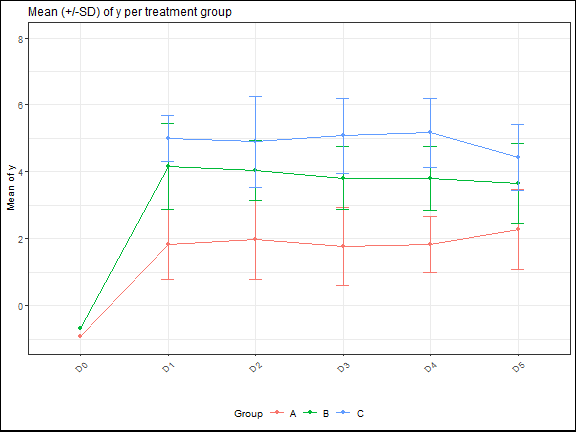### Qualitative graphics

For qualitative statistics, the corresponding graphics will be barplots.

If only the argument `y` is supplied, the corresponding graphic will looks like:

``````# Only one categorical response

tab1=report.quali(data=datafake,y="y_logistic")
plot(tab1,title="Distribution of y (%)",ylab="Percentages",xlab="",ylim=c(0,100))
``````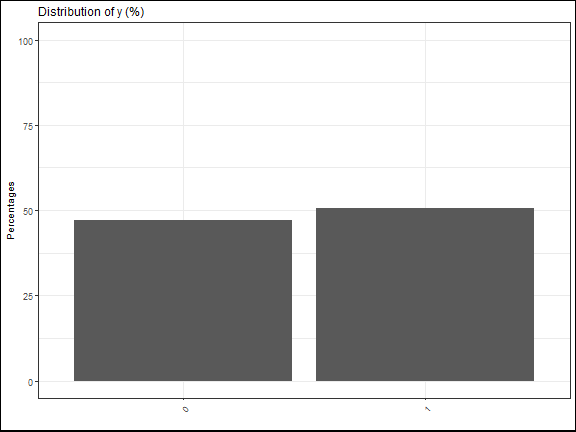If there is one explicative variable, it will be split by color and split on the x-axis:

``````# one categorical response ; one categorical explicative variable

tab1=report.quali(data=datafake,y="y_logistic",x1="GROUP")
plot(tab1,title="Distribution of y per treatment group (%)",
ylab="Percentages",xlab="",ylim=c(0,100),legend.label="Levels of y")
``````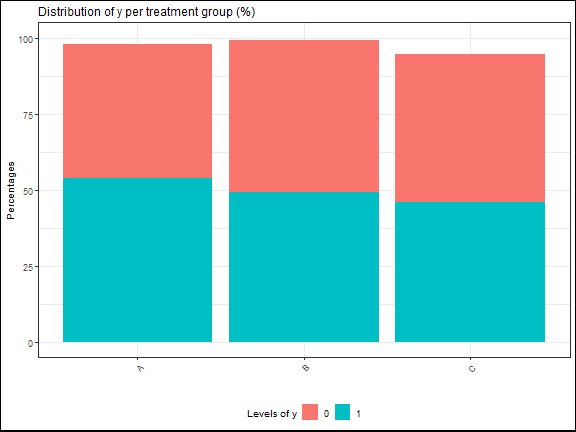If there is two explicative variables, it will be split by color, split on the x-axis and split by panels:

``````# one categorical response ; two categorical explicative variables

tab1=report.quali(data=datafake,y="y_logistic",x1="GROUP",x2="VAR")
plot(tab1,title="Distribution of y per treatment group\nand by level of VAR (%)",
ylab="Percentages",xlab="",ylim=c(0,100),legend.label="Levels of y")
``````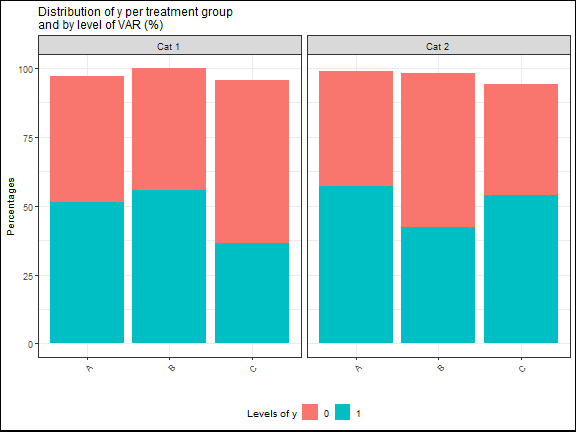### LS-means graphics

For LS-Means graphics, it will be pretty much the same graphics as the graphics of quantitative statistics.

Instead of displaying the standard deviations, it's the 95% confidence intervals that are displayed.

For one explicative variable:

``````# Only one categorical response

mod=lme(y_numeric~GROUP,
random=~1|SUBJID,data=datafake,na.action=na.omit)

test=emmeans(mod,~GROUP)

tab.mod=report.lsmeans(lsm=test)

plot(tab.mod,title="LS-means of y per treatment group",
``````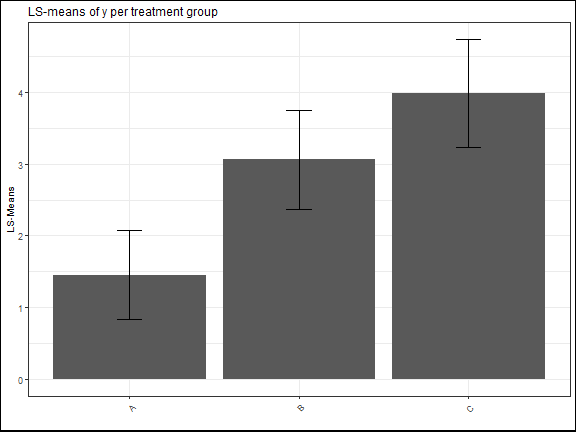With two explicative variables:

``````
# Only one categorical response

mod=lme(y_numeric~baseline+GROUP+TIMEPOINT+GROUP*TIMEPOINT,
random=~1|SUBJID,data=datafake,na.action=na.omit)

test=emmeans(mod,~GROUP|TIMEPOINT)

tab.mod=report.lsmeans(lsm=test)

plot(tab.mod,title="LS-means evolution of y per treatment group\nas a function of time",